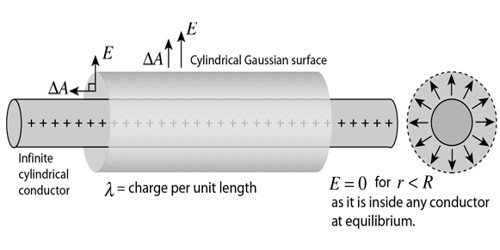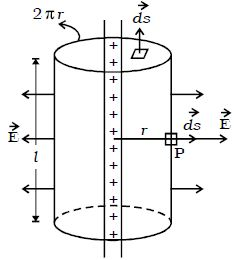# Application of Gauss’s Law: Field due to an Infinite Long Straight Charged Wire

The definitive purpose of Gauss’s law in electrostatics is to find the electric field for a given charge distribution, enclosed by a closed surface. It is the most influential law for electromagnetism.

Gauss’s Law states that – “The total of the electric flux out of a closed surface is equal to the charge enclosed divided by the permittivity.” The closed surface is referred to as the Gaussian surface.

Field due to an Infinite Long Straight Charged Wire (Application of Gauss’s Law):

The first step, the most significant, is to select a Gaussian surface. The proportion of the distribution of charge, then the proportion of the field, suggests a circular cylinder as a Gaussian surface. Assume we have a plain sheet of the infinite extent on which positive charges are equivalently distributed.

Consider a uniformly charged wire of infinite length having a constant linear charge density λ (charge per unit length). Let P be a point at a distance r from the wire (Figure) and E be the electric field at the point P. A cylinder of length l, radius r, closed at each end by plane caps normal to the axis is chosen as the Gaussian surface. Consider a very small area ds on the Gaussian surface.Fig: Infinitely long straight charged wire

By symmetry, the magnitude of the electric field will be the same at all points on the curved surface of the cylinder and directed radially outward. E and ds are in the same direction.

You need to keep in mind that the way of the electric field is thoroughly outward if linear charge density is positive. On the other hand, it will be radically inward if the linear charge density is negative. The top and bottom surfaces of the cylinder lie parallel to the electric field. Thus the angle between area vector and an electric field is 90 degrees and cos θ = 0.

The electric flux (φ) through curved surface = ϐ E ds cos θ

φ = ϐ E ds  [we know, θ = 0; cosθ = 1]

= E (2πrl)

(The surface area of the curved part is 2πrl)

Since E and dsare right angles to each other, the electric flux through the plane caps = 0

so, Total flux through the Gaussian surface, φ = E. (2πrl)

The net charge enclosed by Gaussian surface is, q = λl

By Gauss’s law, E (2πrl) = λl0

or, E = λ / 2πε0r

The direction of electric field E is radially outward if the line charge is positive and inward if the line charge is negative.

Gauss’s Law can be used to solve complex electrostatic problems involving exceptional symmetries like cylindrical, spherical or planar symmetry. We apply Gauss’s Law in the following way:

• Choose a Gaussian surface, such that evaluation of electric field becomes easy
• Make use of symmetry to make problems easier

Remember, it is not necessary that Gaussian surface to coincide with a real surface that is, it can be inside or outside the Gaussian surface.

Note 1: Direction of the electric field will be radially outward if linear charge density is positive and it will be radially inward if linear charge density is negative.

Note 2: We considered only the enclosed charge inside the Gaussian surface.